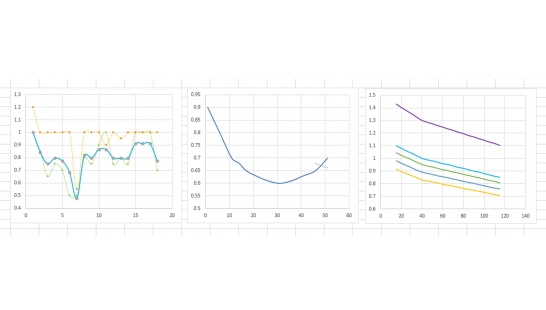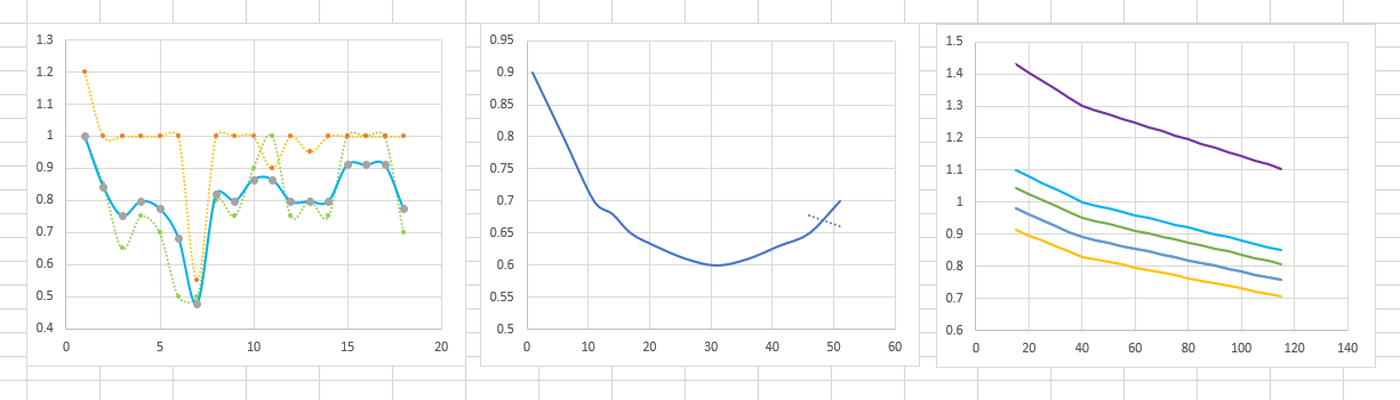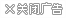|忘记密码

# 天际开瓶器-精金3329
1437 人收藏
5349 次下载
13258 次浏览
02.03

• 详细介绍
• 更新记录
• 玩家评论

1151/5000[技能上限] = 120
[SkillFormulaCaps] = 150

[SkillExpGainMults]

f射手= 0.75
fBlock = 0.80
f共轭= 0.75
fDestruction = 0.90
f迷人= 0.50
fHeavyArmor = 0.80

fLightArmor = 0.80

fOneHanded = 0.90
fPickpocket = 0.80
f恢复= 1.00
fSmithing = 1.00
fSneak = 1.00
fSpeechcraft = 1.00
fTwoHanded = 0.90

[SkillExpGainMults \ CharacterLevel \ XXX]
1 = 1.10
5 = 1.05
8 = 1.02
11 = 1.00

[SkillExpGainMults \ BaseSkillLevel \ XXX]
15 = 1.1
20 = 1.08
25 = 1.06
30 = 1.04
35 = 1.02
40 = 1
45 = 0.99
50 = 0.98
55 = 0.97
60 = 0.96
65 = 0.95
70 = 0.94
75 = 0.93
80 = 0.92
85 = 0.91
90 = 0.9
95 = 0.89
100 = 0.88
105 = 0.87
110 = 0.86
115 = 0.85

[LevelSkillExpMults \ CharacterLevel \ XXX]
1 = 0.90
6 = 0.80
11 = 0.70
14 = 0.68
17 = 0.65
21 = 0.63
26 = 0.61
31 = 0.60
36 = 0.61
41 = 0.63
46 = 0.65
51 = 0.70

[PerksAtLevelUp]
2 = 2.5
3 = 1.5
12 = 1.2
52 = 1.0
82 = 0.5
100 = 1.0
101 = 0.5

[STATAtLevelUp]
2 = 20
3 = 10

[CarryWeightAtHealthLevelUp]
2 = 5
3 = 2

[CarryWeightAtMagickaLevelUp]
2 = 3
3 = 1

[CarryWeightAtStaminaLevelUp]
2 = 10
3 = 5

[传奇技能]
iSkillLevelEnableLegendary = 90
iSkillLevelAfterLegendary = 20——————————————————————————————————————————————————

[SkillCaps] = 120
[SkillFormulaCaps] = 150

[SkillExpGainMults]
fAlchemy     =1.00
fAlteration  =0.80
fMarksman    =0.75
fBlock       =0.80
fConjuration =0.75
fDestruction =0.90
fEnchanting  =0.50
fHeavyArmor  =0.80
fIllusion    =0.75
fLightArmor  =0.80
fLockpicking =1.00
fOneHanded   =0.90
fPickpocket  =0.80
fRestoration =1.00
fSmithing    =1.00
fSneak       =1.00
fSpeechcraft =1.00
fTwoHanded   =0.90

[SkillExpGainMults\CharacterLevel\XXX]
1 =1.10
5 =1.05
8 =1.02
11=1.00

[SkillExpGainMults\BaseSkillLevel\XXX]
15=1.1
20=1.08
25=1.06
30=1.04
35=1.02
40=1
45=0.99
50=0.98
55=0.97
60=0.96
65=0.95
70=0.94
75=0.93
80=0.92
85=0.91
90=0.9
95=0.89
100=0.88
105=0.87
110=0.86
115=0.85

[LevelSkillExpMults\CharacterLevel\XXX]
1= 0.90
6= 0.80
11=0.70
14=0.68
17=0.65
21=0.63
26=0.61
31=0.60
36=0.61
41=0.63
46=0.65
51=0.70

[PerksAtLevelUp]
2   = 2.5
3   = 1.5
12  = 1.2
52  = 1.0
82  = 0.5
100 = 1.0
101 = 0.5

[STATAtLevelUp]
2 = 20
3 = 10

[CarryWeightAtHealthLevelUp]
2 = 5
3 = 2

[CarryWeightAtMagickaLevelUp]
2 = 3
3 = 1

[CarryWeightAtStaminaLevelUp]
2 = 10
3 = 5

[LegendarySkill]
iSkillLevelEnableLegendary = 90
iSkillLevelAfterLegendary = 20

1.版本号: 02.03   更新时间: 2020-04-30 08:57:36

• 感谢
• 支持
• 疑问
• 卖萌
• 关心
• 傲娇

作者精品### 天际开瓶器-精金

Mod大小：2.30KB
上传时间：2020-04-30 08:47:49

### Mod简介：

暂无更多介绍

选择快速回复类型：
• 感谢
• 支持
• 疑问
• 卖萌
• 关心
• 傲娇

综合内容

游戏Mod

单机游戏

手机游戏